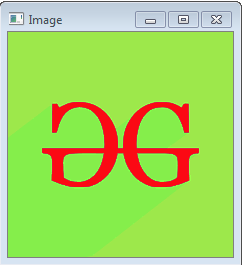# Python OpenCV | cv2.cvtColor() method

• Difficulty Level : Basic
• Last Updated : 18 Oct, 2019

OpenCV-Python is a library of Python bindings designed to solve computer vision problems. `cv2.cvtColor()` method is used to convert an image from one color space to another. There are more than 150 color-space conversion methods available in OpenCV. We will use some of color space conversion codes below.

Syntax: cv2.cvtColor(src, code[, dst[, dstCn]])

Parameters:
src: It is the image whose color space is to be changed.
code: It is the color space conversion code.
dst: It is the output image of the same size and depth as src image. It is an optional parameter.
dstCn: It is the number of channels in the destination image. If the parameter is 0 then the number of the channels is derived automatically from src and code. It is an optional parameter.

Return Value: It returns an image.

Image used for all the below examples:Example #1:

 `# Python program to explain cv2.cvtColor() method  ` `  `  `# importing cv2  ` `import` `cv2  ` `  `  `# path  ` `path ``=` `r``'C:\Users\Administrator\Desktop\geeks.png'` `  `  `# Reading an image in default mode ` `src ``=` `cv2.imread(path) ` `  `  `# Window name in which image is displayed ` `window_name ``=` `'Image'` ` `  `# Using cv2.cvtColor() method ` `# Using cv2.COLOR_BGR2GRAY color space ` `# conversion code ` `image ``=` `cv2.cvtColor(src, cv2.COLOR_BGR2GRAY ) ` ` `  `# Displaying the image  ` `cv2.imshow(window_name, image) `

Output:Example #2:
Using HSV color space. HSV color space is mostly used for object tracking.

 `# Python program to explain cv2.cvtColor() method  ` `  `  `# importing cv2  ` `import` `cv2  ` `  `  `# path  ` `path ``=` `r``'C:\Users\Administrator\Desktop\geeks.png'` `  `  `# Reading an image in default mode ` `src ``=` `cv2.imread(path) ` `  `  `# Window name in which image is displayed ` `window_name ``=` `'Image'` ` `  `# Using cv2.cvtColor() method ` `# Using cv2.COLOR_BGR2HSV color space ` `# conversion code ` `image ``=` `cv2.cvtColor(src, cv2.COLOR_BGR2HSV ) ` ` `  `# Displaying the image  ` `cv2.imshow(window_name, image) `

Output:My Personal Notes arrow_drop_up
Recommended Articles
Page :